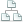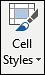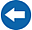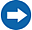Home >Tips >Excel 2016 from Scratch >Absolute and relative referencesSite map

# Excel 2016 from Scratch Exercise Sum, Average, Max and Min

## An Excel tutorial by Peter KalmstromIn this demo in the Excel 2016 from Scratch series, Peter Kalmstrom talks some more about how to find maximum and minumum values and calculate averages and sums.

As an example Peter uses a shipping company that needs statistics on how many passenges embark each month in different ports. The shipowners want to know the total sum for each month and port and also the average, maximum and minimum for each month and port.

### Exercise

If you want to try the exercise yourself, please download the Excel file Peter uses in the demo!

### Content

This is what Peter shows in the demo below:

• How to use the AutoSum button to calculate a sum.
• How to use the AutoSum button to calculate an average.
• How to use the AutoSum button to find a maximum.
• How to use the AutoSum button to find a minimum.
• How to fill a formula into other cells.
• How to write a formula that calculates a sum.
• How to write a formula that calculates an average.
• How to write a formula that finds the maximum.
• How to write a formula that finds the minimum.
• How to remove decimals.
•How to use the predefined Excel Cell Styles to give different formatting to different cells, depending on their content.
Peter uses Excel 2016 for his demo, but the Excel basics are the same for earlier versions of Excel.